# spike_train_correlation - Spike train correlation¶

This modules provides functions to calculate correlations between spike trains.

elephant.spike_train_correlation.corrcoef(binned_sts, binary=False)[source]

Calculate the NxN matrix of pairwise Pearson’s correlation coefficients between all combinations of N binned spike trains.

For each pair of spike trains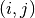, the correlation coefficient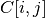is obtained by binningand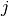at the desired bin size. Let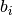and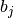denote the binary vectors and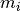and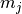their respective averages. Then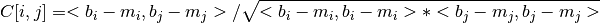where <..,.> is the scalar product of two vectors.

For an input of n spike trains, a n x n matrix is returned. Each entry in the matrix is a real number ranging between -1 (perfectly anti-correlated spike trains) and +1 (perfectly correlated spike trains).

If binary is True, the binned spike trains are clipped to 0 or 1 before computing the correlation coefficients, so that the binned vectorsandare binary.

Parameters: binned_sts : elephant.conversion.BinnedSpikeTrain A binned spike train containing the spike trains to be evaluated. binary : bool, optional If True, two spikes of a particular spike train falling in the same bin are counted as 1, resulting in binary binned vectors. If False, the binned vectorscontain the spike counts per bin. Default: False C : ndarrray The square matrix of correlation coefficients. The element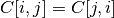is the Pearson’s correlation coefficient between binned_sts[i] and binned_sts[j]. If binned_sts contains only one SpikeTrain, C=1.0.

Notes

• The spike trains in the binned structure are assumed to all cover the complete time span of binned_sts [t_start,t_stop).

Examples

Generate two Poisson spike trains

>>> from elephant.spike_train_generation import homogeneous_poisson_process
>>> st1 = homogeneous_poisson_process(
rate=10.0*Hz, t_start=0.0*s, t_stop=10.0*s)
>>> st2 = homogeneous_poisson_process(
rate=10.0*Hz, t_start=0.0*s, t_stop=10.0*s)


Calculate the correlation matrix.

>>> from elephant.conversion import BinnedSpikeTrain
>>> cc_matrix = corrcoef(BinnedSpikeTrain([st1, st2], binsize=5*ms))


The correlation coefficient between the spike trains is stored in cc_matrix[0,1] (or cc_matrix[1,0]).

elephant.spike_train_correlation.covariance(binned_sts, binary=False)[source]

Calculate the NxN matrix of pairwise covariances between all combinations of N binned spike trains.

For each pair of spike trains, the covarianceis obtained by binningandat the desired bin size. Letanddenote the binary vectors andandtheir respective averages. Then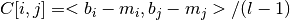where <..,.> is the scalar product of two vectors.

For an input of n spike trains, a n x n matrix is returned containing the covariances for each combination of input spike trains.

If binary is True, the binned spike trains are clipped to 0 or 1 before computing the covariance, so that the binned vectorsandare binary.

Parameters: binned_sts : elephant.conversion.BinnedSpikeTrain A binned spike train containing the spike trains to be evaluated. binary : bool, optional If True, two spikes of a particular spike train falling in the same bin are counted as 1, resulting in binary binned vectors. If False, the binned vectorscontain the spike counts per bin. Default: False C : ndarrray The square matrix of covariances. The elementis the covariance between binned_sts[i] and binned_sts[j].

Notes

• The spike trains in the binned structure are assumed to all cover the complete time span of binned_sts [t_start,t_stop).

Examples

Generate two Poisson spike trains

>>> from elephant.spike_train_generation import homogeneous_poisson_process
>>> st1 = homogeneous_poisson_process(
rate=10.0*Hz, t_start=0.0*s, t_stop=10.0*s)
>>> st2 = homogeneous_poisson_process(
rate=10.0*Hz, t_start=0.0*s, t_stop=10.0*s)


Calculate the covariance matrix.

>>> from elephant.conversion import BinnedSpikeTrain
>>> cov_matrix = covariance(BinnedSpikeTrain([st1, st2], binsize=5*ms))


The covariance between the spike trains is stored in cc_matrix[0,1] (or cov_matrix[1,0]).

elephant.spike_train_correlation.cross_correlation_histogram(binned_st1, binned_st2, window='full', border_correction=False, binary=False, kernel=None, method='speed', cross_corr_coef=False)[source]

Computes the cross-correlation histogram (CCH) between two binned spike trains binned_st1 and binned_st2.

Parameters: binned_st1, binned_st2 : BinnedSpikeTrain Binned spike trains to cross-correlate. The two spike trains must have same t_start and t_stop window : string or list of integer (optional) ‘full’: This returns the crosscorrelation at each point of overlap, with an output shape of (N+M-1,). At the end-points of the cross-correlogram, the signals do not overlap completely, and boundary effects may be seen. ‘valid’: Mode valid returns output of length max(M, N) - min(M, N) + 1. The cross-correlation product is only given for points where the signals overlap completely. Values outside the signal boundary have no effect. list of integer (window=minimum lag, window=maximum lag): The entries of window are two integers representing the left and right extremes (expressed as number of bins) where the crosscorrelation is computed Default: ‘full’ border_correction : bool (optional) whether to correct for the border effect. If True, the value of the CCH at bin b (for b=-H,-H+1, …,H, where H is the CCH half-length) is multiplied by the correction factor: (H+1)/(H+1-|b|), which linearly corrects for loss of bins at the edges. Default: False binary : bool (optional) whether to binary spikes from the same spike train falling in the same bin. If True, such spikes are considered as a single spike; otherwise they are considered as different spikes. Default: False. kernel : array or None (optional) A one dimensional array containing an optional smoothing kernel applied to the resulting CCH. The length N of the kernel indicates the smoothing window. The smoothing window cannot be larger than the maximum lag of the CCH. The kernel is normalized to unit area before being applied to the resulting CCH. Popular choices for the kernel are normalized boxcar kernel: numpy.ones(N) hamming: numpy.hamming(N) hanning: numpy.hanning(N) bartlett: numpy.bartlett(N) If None is specified, the CCH is not smoothed. Default: None method : string (optional) Defines the algorithm to use. “speed” uses numpy.correlate to calculate the correlation between two binned spike trains using a non-sparse data representation. Due to various optimizations, it is the fastest realization. In contrast, the option “memory” uses an own implementation to calculate the correlation based on sparse matrices, which is more memory efficient but slower than the “speed” option. Default: “speed” cross_corr_coef : bool (optional) Normalizes the CCH to obtain the cross-correlation coefficient function ranging from -1 to 1 according to Equation (5.10) in “Analysis of parallel spike trains”, 2010, Gruen & Rotter, Vol 7 cch : AnalogSignal Containing the cross-correlation histogram between binned_st1 and binned_st2. The central bin of the histogram represents correlation at zero delay. Offset bins correspond to correlations at a delay equivalent to the difference between the spike times of binned_st1 and those of binned_st2: an entry at positive lags corresponds to a spike in binned_st2 following a spike in binned_st1 bins to the right, and an entry at negative lags corresponds to a spike in binned_st1 following a spike in binned_st2. To illustrate this definition, consider the two spike trains: binned_st1: 0 0 0 0 1 0 0 0 0 0 0 binned_st2: 0 0 0 0 0 0 0 1 0 0 0 Here, the CCH will have an entry of 1 at lag h=+3. Consistent with the definition of AnalogSignals, the time axis represents the left bin borders of each histogram bin. For example, the time axis might be: np.array([-2.5 -1.5 -0.5 0.5 1.5]) * ms bin_ids : ndarray of int Contains the IDs of the individual histogram bins, where the central bin has ID 0, bins the left have negative IDs and bins to the right have positive IDs, e.g.,: np.array([-3, -2, -1, 0, 1, 2, 3])
elephant.spike_train_correlation.spike_time_tiling_coefficient(spiketrain_1, spiketrain_2, dt=array(0.005) * s)[source]

Calculates the Spike Time Tiling Coefficient (STTC) as described in (Cutts & Eglen, 2014) following Cutts’ implementation in C. The STTC is a pairwise measure of correlation between spike trains. It has been proposed as a replacement for the correlation index as it presents several advantages (e.g. it’s not confounded by firing rate, appropriately distinguishes lack of correlation from anti-correlation, periods of silence don’t add to the correlation and it’s sensitive to firing patterns).

The STTC is calculated as follows: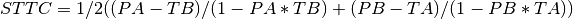Where PA is the proportion of spikes from train 1 that lie within [-dt, +dt] of any spike of train 2 divided by the total number of spikes in train 1, PB is the same proportion for the spikes in train 2; TA is the proportion of total recording time within [-dt, +dt] of any spike in train 1, TB is the same proportion for train 2. For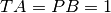the resulting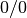is replaced with, since every spike from the train with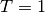is within [-dt, +dt] of a spike of the other train.

This is a Python implementation compatible with the elephant library of the original code by C. Cutts written in C and avaiable at: (https://github.com/CCutts/Detecting_pairwise_correlations_in_spike_trains/blob/master/spike_time_tiling_coefficient.c)

Parameters: spiketrain_1, spiketrain_2: neo.Spiketrain objects to cross-correlate. Must have the same t_start and t_stop. dt: Python Quantity. The synchronicity window is used for both: the quantification of the proportion of total recording time that lies [-dt, +dt] of each spike in each train and the proportion of spikes in spiketrain_1 that lies [-dt, +dt] of any spike in spiketrain_2. Default : 0.005 * pq.s index: float The spike time tiling coefficient (STTC). Returns np.nan if any spike train is empty.

References

Cutts, C. S., & Eglen, S. J. (2014). Detecting Pairwise Correlations in Spike Trains: An Objective Comparison of Methods and Application to the Study of Retinal Waves. Journal of Neuroscience, 34(43), 14288–14303.